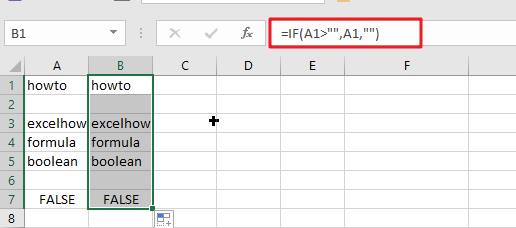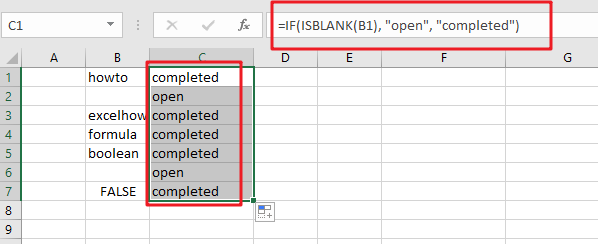# Check If a Cell is Blank or Empty

The article demonstrates how to check if a range of cells is blank or empty by ISBLANK and other functions to recognize empty cells in Excel and take appropriate action based on their status.

There are several circumstances in which you need to determine if a cell is empty or not. If a cell is blank, for example, you may want to add, count, copy a value from another cell, or do nothing. ISBLANK is the appropriate function to employ in these situations, occasionally alone, but most often in conjunction with other Excel functions.

## ISBLANK in Excel – a few points to keep in mind

The critical item to remember is that the ISBLANK function in Excel recognizes actually empty cells, that is, cells that contain nothing: no spaces, no tabs, no carriage returns, or anything else that looks blank in a view.

ISBLANK returns FALSE for a cell that seems to be blank but is not. If a cell includes any of the following, this behavior occurs:

`=IF(A1>"", A1, "") //yields an empty string`Imported zero-length string from an external database or as a consequence of a copy/paste operation.

Spaces, apostrophes, non-breaking spaces (&nbsp);, linefeeds, and other non-printing characters are permitted.

## How to utilize the ISBLANK function in Excel

To obtain a better grasp of the ISBLANK function‘s capabilities, consider these practical applications.

### If a cell is blank in Excel, then

Because Microsoft Excel does not have an IFBLANK function, you must use IF and ISBLANK to test a cell and execute an action if it is empty.

The general version is as follows:

`=IF(ISBLANK(B1), "open", "completed")`To demonstrate, let’s verify whether a cell in column B (delivery date) has any data. If the cell is empty, output “Open”; if it is not empty, produce “Completed”.

Please keep in mind that the ISBLANK function only returns cells that are completely blank. ISBLANK returns FALSE if a cell contains anything invisible to the human eye, such as a zero-length string.

### If any cell in range is blank, then do something

There are many techniques to check a range of cells in Microsoft Excel for empty cells. We will use an IF statement to output one value if the range has at least one empty cell and another value if the range contains no empty cells. The logical test is performed by calculating the total number of empty cells in the range and then determining if the count is larger than zero. This may be accomplished via the use of the COUNTBLANK or COUNTIF functions.

### Related Functions

• Excel ISBLANK function
The Excel ISBLANK function returns TRUE if the value is blank or null.The syntax of the ISBLANK function is as below:= ISBLANK (value)…
• Excel IF function
The Excel IF function perform a logical test to return one value if the condition is TRUE and return another value if the condition is FALSE. The IF function is a build-in function in Microsoft Excel and it is categorized as a Logical Function.The syntax of the IF function is as below:= IF (condition, [true_value], [false_value])….
• Excel COUNTIF function
The Excel COUNTIF function will count the number of cells in a range that meet a given criteria. This function can be used to count the different kinds of cells with number, date, text values, blank, non-blanks, or containing specific characters.etc.= COUNTIF (range, criteria)…
• Excel COUNTBLANK function
The Excel COUNTBLANK function use to count the number of empty cells in a range of cells. So you can use the COUNTBLANK function to get the number of blank cells in a given range in Excel.The syntax of the COUNTBLANK function is as below:= COUNTBLANK (range)…
Related Posts

Calculate Days Open in Excel

If you want to know how to Calculate days in Excel, there are some formulas that you can use to do so. For example, you can use the DAYS function in Excel to find the number of days between two ...

Add Row Numbers And Skip Blanks in Excel

Do you ever have to input a list of numbers into a spreadsheet, and some of the cells are blank? It can be difficult to keep track of what number is in which cell when you have to scroll up ...

If Value is Greater Than A Certain Value

IF function is frequently used in Excel worksheet to return you expect “true value” or “false value” based on the logical test result. If you want to see if a value in one cell is greater than a specific value, ...

If Cell is Not Blank

IF function is frequently used in Excel worksheet to return you expect “true value” or “false value” based on the result of created logical test. If you want to see if a cell is blank or not, and leave some ...

If Cell is Blank

IF function is frequently used in Excel worksheet to return you expect “true value” or “false value” based on the result of created logical test. If you want to see if a cell is blank or not, and leave some ...

If Cell Equals Certain Text String

IF function is frequently used in Excel worksheet to return you expect “true value” or “false value” based on the result of created logical test. If you want to see if cell equals a certain text string like “Win”, you ...

If Cell Contains Either Text1 or Text2

IF function is frequently used in Excel worksheet to return “true value” or “false value” based on the logical test result. If you want to see if cell contains certain substring1 like “abc” or substring2 like “def”, and returns true ...

If Cell Contains Certain Text OR Equals Certain Text

IF cell equals certain text IF function is frequently used in Excel worksheet to return “true value” or “false value” based on the logical test result. If you want to test values to see if they equal certain text like ...

Fix #N/A Error For VLOOKUP From Another Sheet

This post will show you how to fix the #N/A error why it occurs when you extract values from another sheet using VLOOKUP function in Excel 2016,2013,2010 or other Excel versions. How can you correct a #N/A error in VLOOKUP ...

How to Average Only Positive or Negative Numbers of a Range

Suppose both positive numbers and negative numbers exist in a table. If we want to know the average of only positive numbers in this table, we can create a formula to get average of all positive numbers with all negative ...

Sidebar